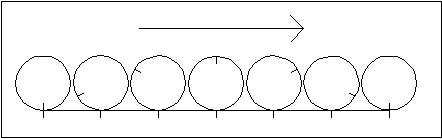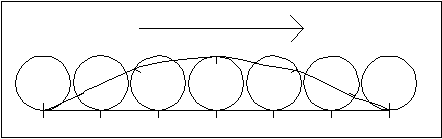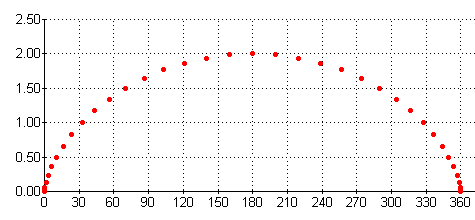## The Cycloidal Curve

In order to simulate the rolling action of two discs, we must consider the path traced by a point on the edge (circumference) of one of the discs.

First consider the path traced by a point on the edge of a circle that rotates in a stationary position. In this drawing, the point is where the small line meets the edge of the circle. The path is obviously that of a circle.Now consider the path traced by this point when the circle is rolling on a horizontal plane. (I have deliberately exaggerated the horizontal movement of the circle in the next drawing to illustrate more clearly the path of the point on the circle.)If you draw a line to trace the path of the point as the circle rotates, the result is called a Cycloidal Curve.This curve is inaccurate in its shape because of the way I created these drawings, so below is a much more accurate Cycloidal Curve, generated in a chart of the horizontal and vertical coordinates of the path of the point as the circle rotates. The chart is used here to create the graph below (with Rotation in Degrees on the X axis and Displacement in Inches on the Y axis).Go To Epicycloidal Curve

Clock Repair Main Page
Escapements in Motion